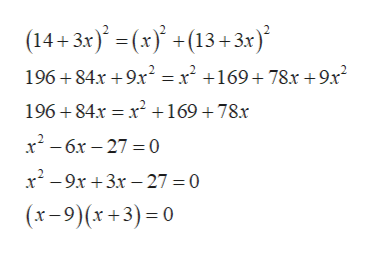# The length of the longer leg of a right triangle is 13inchesmore than three times the length of the shorter leg. The length of the hypotenuse is 14inchesmore than three times the length of the shorter leg. Find the side lengths of the triangle.

Question
57 views

The length of the longer leg of a right triangle is
13
inches

more than three times the length of the shorter leg. The length of the hypotenuse is
14
inches

more than three times the length of the shorter leg. Find the side lengths of the triangle.

check_circle

Step 1

Let the length of shorter leg be x.

Then the length of the longer leg will be 13+3x.

The length of the hypotenuse will be 14+3x.

According to the pythogrus theorem,

Step 2

Substitute  the values.help_outlineImage Transcriptionclose(14+3x(x (13+3x) 196+84x +9x2 x2 +169+ 78x +9x2 196 84x x2 +169 +78x x26x27 0 x-9x3x 27 = 0 (x-9)(x+3) 0 fullscreen
Step 3

Equate each fa...

### Want to see the full answer?

See Solution

#### Want to see this answer and more?

Solutions are written by subject experts who are available 24/7. Questions are typically answered within 1 hour.*

See Solution
*Response times may vary by subject and question.
Tagged in

### Algebra